# Discrete Mathematics - Spanning Trees

A spanning tree of a connected undirected graph $G$ is a tree that minimally includes all of the vertices of $G$. A graph may have many spanning trees.

### Example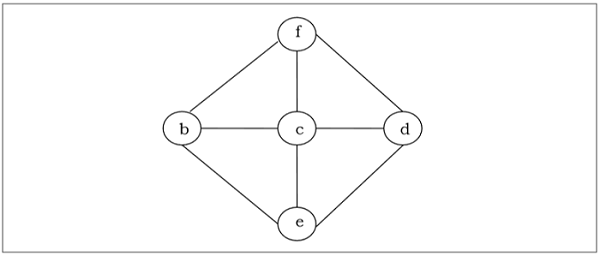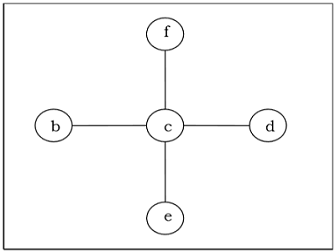## Minimum Spanning Tree

A spanning tree with assigned weight less than or equal to the weight of every possible spanning tree of a weighted, connected and undirected graph $G$, it is called minimum spanning tree (MST). The weight of a spanning tree is the sum of all the weights assigned to each edge of the spanning tree.

### Example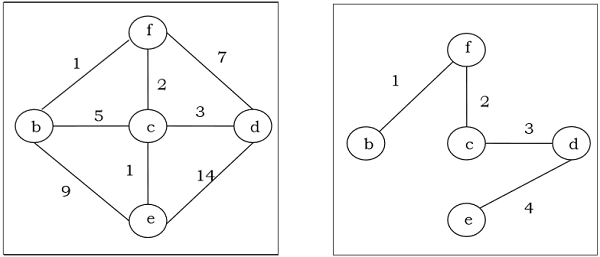## Kruskal's Algorithm

Kruskal's algorithm is a greedy algorithm that finds a minimum spanning tree for a connected weighted graph. It finds a tree of that graph which includes every vertex and the total weight of all the edges in the tree is less than or equal to every possible spanning tree.

### Algorithm

Step 1 − Arrange all the edges of the given graph $G (V,E)$ in ascending order as per their edge weight.

Step 2 − Choose the smallest weighted edge from the graph and check if it forms a cycle with the spanning tree formed so far.

Step 3 − If there is no cycle, include this edge to the spanning tree else discard it.

Step 4 − Repeat Step 2 and Step 3 until $(V-1)$ number of edges are left in the spanning tree.

Problem

Suppose we want to find minimum spanning tree for the following graph G using Kruskal’s algorithm.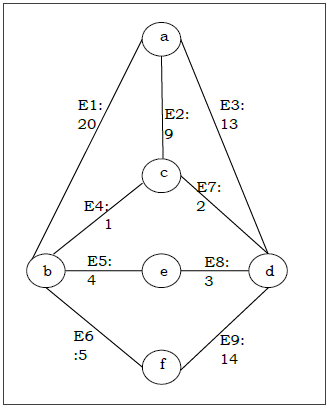Solution

From the above graph we construct the following table −

Edge No. Vertex Pair Edge Weight
E1 (a, b) 20
E2 (a, c) 9
E3 (a, d) 13
E4 (b, c) 1
E5 (b, e) 4
E6 (b, f) 5
E7 (c, d) 2
E8 (d, e) 3
E9 (d, f) 14

Now we will rearrange the table in ascending order with respect to Edge weight −

Edge No. Vertex Pair Edge Weight
E4 (b, c) 1
E7 (c, d) 2
E8 (d, e) 3
E5 (b, e) 4
E6 (b, f) 5
E2 (a, c) 9
E3 (a, d) 13
E9 (d, f) 14
E1 (a, b) 20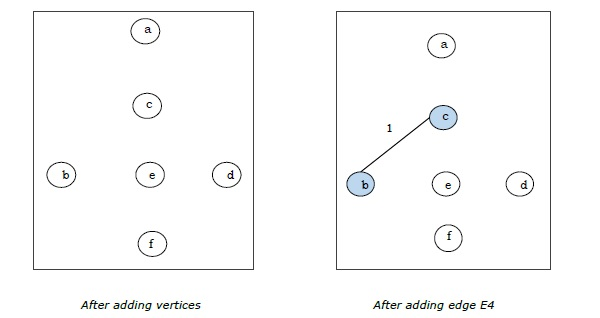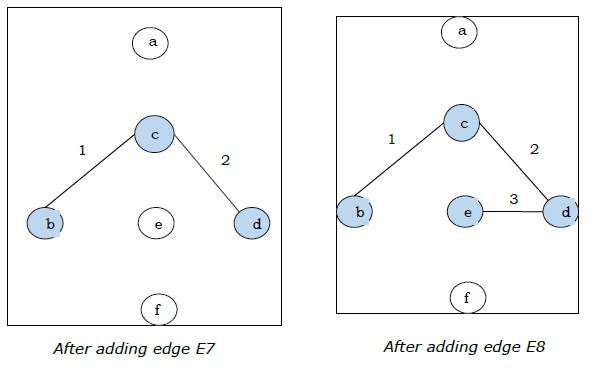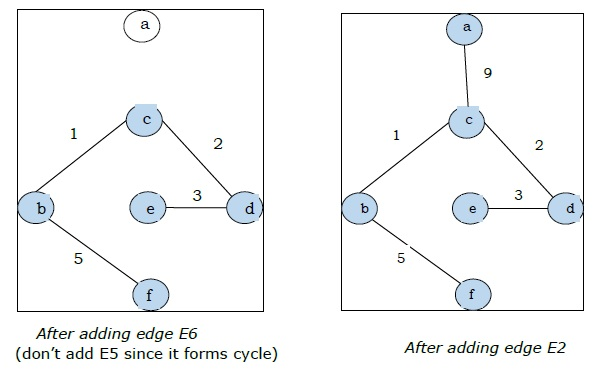Since we got all the 5 edges in the last figure, we stop the algorithm and this is the minimal spanning tree and its total weight is $(1 + 2 + 3 + 5 + 9) = 20$.

## Prim's Algorithm

Prim's algorithm, discovered in 1930 by mathematicians, Vojtech Jarnik and Robert C. Prim, is a greedy algorithm that finds a minimum spanning tree for a connected weighted graph. It finds a tree of that graph which includes every vertex and the total weight of all the edges in the tree is less than or equal to every possible spanning tree. Prim’s algorithm is faster on dense graphs.

### Algorithm

• Initialize the minimal spanning tree with a single vertex, randomly chosen from the graph.

• Repeat steps 3 and 4 until all the vertices are included in the tree.

• Select an edge that connects the tree with a vertex not yet in the tree, so that the weight of the edge is minimal and inclusion of the edge does not form a cycle.

• Add the selected edge and the vertex that it connects to the tree.

Problem

Suppose we want to find minimum spanning tree for the following graph G using Prim’s algorithm.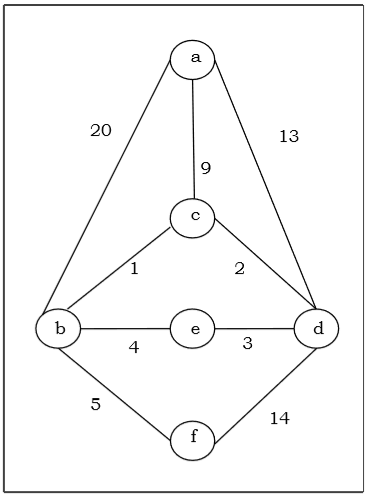Solution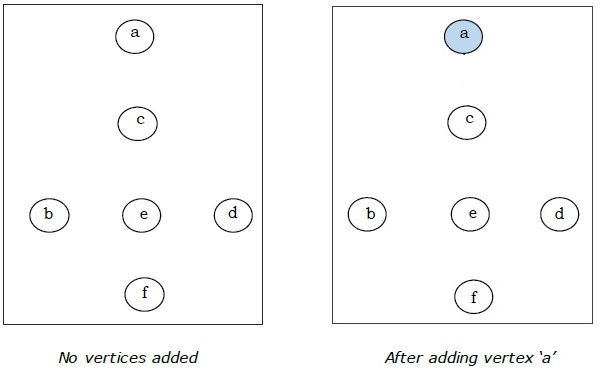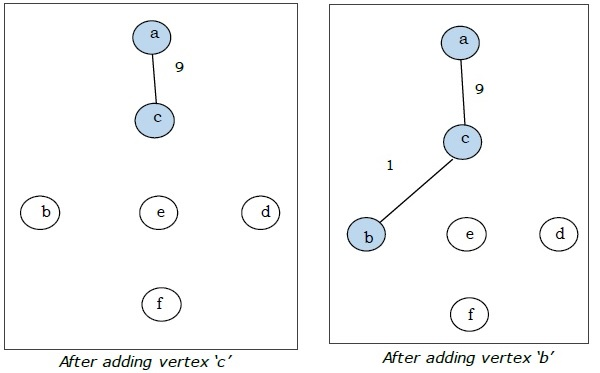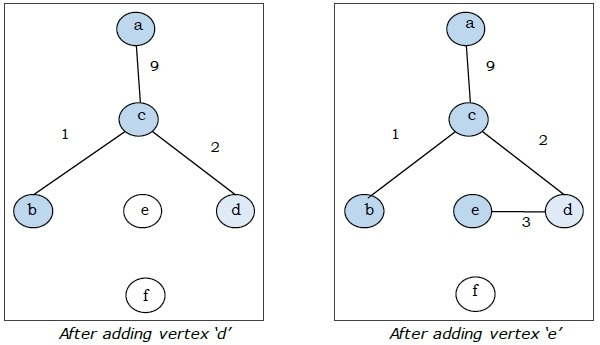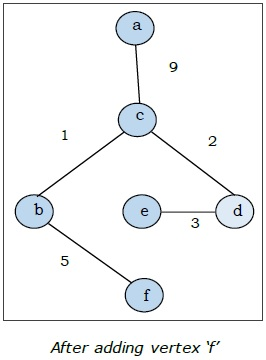This is the minimal spanning tree and its total weight is $(1 + 2 + 3 + 5 + 9) = 20$.